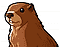# mORMot Open Source

You are not logged in.

## #1 2015-08-11 09:56:17

lele9
Member
Registered: 2011-10-28
Posts: 170

### TTimeLog and Javascript client

Hi,
i'm writing a javascript client that get data with a query.
now i should write a condition on a TTimeLog field but i don't know how to convert a Date to TTimeLog in javascript.
Any idea?
thanks,
Emanuele.

Offline

## #2 2015-08-11 12:56:21

abFrom: France
Registered: 2010-06-21
Posts: 13,763
Website

### Re: TTimeLog and Javascript client

Just encode a JavaScript date with the bit-shifted values from the TTimeLog integer content.

``````function TTimeLogBits.Year: Integer;
begin
result := (Value shr (6+6+5+5+4)) and 4095;
end;

function TTimeLogBits.Month: Integer;
begin
result := 1+(Int64Rec(Value).Lo shr (6+6+5+5)) and 15;
end;

function TTimeLogBits.Day: Integer;
begin
result := 1+(Int64Rec(Value).Lo shr (6+6+5)) and 31;
end;

function TTimeLogBits.Hour: Integer;
begin
result := (Int64Rec(Value).Lo shr (6+6)) and 31;
end;

function TTimeLogBits.Minute: Integer;
begin
result := (Int64Rec(Value).Lo shr 6) and 63;
end;

function TTimeLogBits.Second: Integer;
begin
result := Int64Rec(Value).Lo and 63;
end;``````

Offline

## #3 2015-08-11 13:59:39

lele9
Member
Registered: 2011-10-28
Posts: 170

### Re: TTimeLog and Javascript client

hi ab,
excuse me but maybe i explained wrong or i don't understand what do you mean.
i retrieve data from a javascript client page (that use the mormotClient.js written from Esmond, thanks!).
I need to query on TTimeLog field and so, i think, i need to encode a DateTime javascript variable to TTimeLog.
how to do?
thanks

Offline

## #4 2015-08-11 14:51:35

zed
Member
From: Belarus
Registered: 2015-02-26
Posts: 103

### Re: TTimeLog and Javascript client

Take a look to the mORMot sources (SynCommons.pas):

``````// bits: S=0..5 M=6..11 H=12..16 D=17..21 M=22..25 Y=26..38
// size: S=6 M=6  H=5  D=5  M=4  Y=12
// i.e. S<64 M<64 H<32 D<32 M<16 Y<4096: power of 2 -> use fast shl/shr

procedure TTimeLogBits.From(Y, M, D, HH, MM, SS: cardinal);
begin
inc(HH,D shl 5+M shl 10+Y shl 14-(1 shl 5+1 shl 10));
Value := SS+MM shl 6+Int64(HH) shl 12;
end;``````

Offline

## #5 2015-08-11 15:05:23

lele9
Member
Registered: 2011-10-28
Posts: 170

### Re: TTimeLog and Javascript client

i looked and i write this javascript code but the number dont seems a ttimelog...

``````var timeLog = 0 + (day << 5) + (month << 10) + (year << 14) - ((1 << 5) + (1 << 10));
timeLog = (timeLog << 12);``````

NB i need date only
where i mistake?

Offline

## #6 2015-08-11 16:09:03

zed
Member
From: Belarus
Registered: 2015-02-26
Posts: 103

### Re: TTimeLog and Javascript client

I just googled that JavaScript bit-level operations are limited to 32 bit. You can use some library to work with 64-bit integers in JS, for example: http://google.github.io/closure-library … _Long.html

Offline

## #7 2015-08-11 19:29:53

abFrom: France
Registered: 2010-06-21
Posts: 13,763
Website

Offline

## #8 2015-08-12 06:12:19

lele9
Member
Registered: 2011-10-28
Posts: 170

### Re: TTimeLog and Javascript client

``````var timeLog = 0 + (day * 5) + (month * 10) + (year * 14) - ((1 * 5) + (1 * 10));
timeLog = (timeLog * 12);``````

like this?

Offline

## #9 2015-08-12 06:24:33

abFrom: France
Registered: 2010-06-21
Posts: 13,763
Website

### Re: TTimeLog and Javascript client

Using powers of two, of course!

Offline

## #10 2015-08-12 06:32:36

lele9
Member
Registered: 2011-10-28
Posts: 170

### Re: TTimeLog and Javascript client

ooops sorry, now i understand!

``````var temp = 0 + (day * Math.pow(2,5)) + (month * Math.pow(2,10)) + (year * Math.pow(2,14)) - ((1 * Math.pow(2,5)) + (1 * Math.pow(2,10)));
var timeLog = temp * Math.pow(2,12);``````

this javascript code seems to work!

Offline

## #11 2015-08-12 15:09:06

lele9
Member
Registered: 2011-10-28
Posts: 170

### Re: TTimeLog and Javascript client

any idea to write a javascript TimeLogToDateTime function?

Offline

## #12 2015-08-13 08:20:25

lele9
Member
Registered: 2011-10-28
Posts: 170

### Re: TTimeLog and Javascript client

for other user, i hope can help.
excuse my poor javascript.

``````function TimeLogFromDateTime(dataText) {
var giorno = new Date(dataText).getDate();
var mese = new Date(dataText).getMonth();
mese++;
var anno = new Date(dataText).getFullYear();
var temp = 0 + (giorno * Math.pow(2,5)) + (mese * Math.pow(2,10)) + (anno * Math.pow(2,14)) - ((1 * Math.pow(2,5)) + (1 * Math.pow(2,10)));
var result = temp * Math.pow(2,12);
return result;
}

function TimeLogToDateTime(Value) {
var result = new Date();
var Y = Math.round(Value / Math.pow(2,(6+6+5+5+4)) & 4095);
var M = 1+((Value) / Math.pow(2,(6+6+5+5))) & 15;
var D = 1+((Value) / Math.pow(2,(6+6+5))) & 31;
result.setFullYear(Y);
result.setMonth(M-1);
result.setDate(D);
result.setHours(0);
result.setMinutes(0);
result.setSeconds(0);
result.setMilliseconds(0);
if (Value & (1 * Math.pow(2,(6+6+5))-1) != 0) {
var HH = ((Value) / Math.pow(2,(6+6))) & 31;
var MM = ((Value) / Math.pow(2,6)) & 63;
var SS = (Value) & 63;
result.setHours(HH);
result.setMinutes(MM);
result.setSeconds(SS);
result.setMilliseconds(0);
}
return result;
}``````

Offline

## #13 2015-08-13 18:03:48

ramersonw
Member
From: Brazil
Registered: 2015-07-16
Posts: 6

### Re: TTimeLog and Javascript client

Thanks lele9. I was looking for this too...

Offline